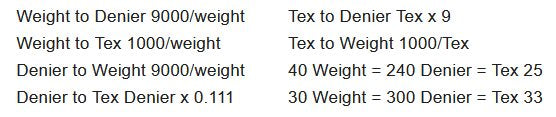### Sewing Machine Thread Sizing Guide

Thread Size Measurement: Two primary methods, length and weight.

Weight

In weight measurements, higher numbers reflect finer or lighter threads. The “weight” of a particular type of thread refers to the length of a given weight of thread. Dividing the length of thread by a set weight derives the exact measurement of a thread weight. A thread is labeled 40 wt. because one gram is 40 meters long. A thread is labeled 30 wt. because one gram is 30 meters long. A 30-wt. thread is a “heavier” thread, meaning a thicker cross section, because a much shorter thread weighs the same as the 40 wt.

Length

In length measurements, higher numbers reflect thicker or heavier threads. “Denier” refers to the weight in grams of 9000 meters of thread. If 9000 meters weighs 1120 grams, it is a 120 d thread. Most embroidery threads are 120/2, which equals 2 strands of 120-denier thread for a 240 denier total.

“Tex”

Refers to the weight in grams of 1000 meters of thread. If 1000 meters weighs 25 grams, it is Tex 25.

Rule of Thumb

40 Wt. = 240 denier = Tex 25. All work in a size 75/11 needle. SCHMETZ advises that the diameter of the eye of the needle should be 40% larger than the diameter of the thread. Using the rule of thumb, consider a larger needle when using threads heavier than 40wt/240denier/tex25. Consider using a smaller needle when using finer threads.

### Conversion FactorsThe Physical Dimension of a Thread Affects:

Both top and bobbin tensions — Changing the thread physically changes the tension. When thread size is changed, the upper and lower tensions should be checked.

Thread displacement — Too many thread fibers in a set space make the fabric pucker. Reduce field density, scale pattern or increase stitch length.

Needle selection — Eye of the needle should be 40% larger than the diameter of the thread. When going to a larger size thread, a larger needle should be used.